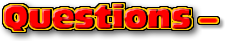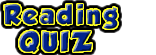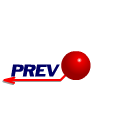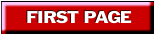ChaptersPage 6
This text is meant to accompany class discussions. It is not everything there is to know about the basics of electricity. It is meant as a  prep for class. More detailed notes and examples are given in the class notes, presentations, and demonstrations (click here.)Click for the questions that go with this reading
Adding and Subtracting Vectors by Components

A component is the a smaller piece of a bigger body. These smaller pieces add up to create something. for example, in general terms your car has a body, power source, braking system, and an electrical system. These four items add up to equal your car. The human body has 206 bones (if the sternum is considered one bone instead of three.) These 206 bones add up to be the skeleton.

When discussing components of vectors the "add" literally means to perform a mathematical summation operation on them.

Part 1 of 3

Part 2 of 3

Part 3 of 3

Example
• Question
• Answer
• Your Paper
• Video Solution

Using the method of components, add up the following vectors. (Use the angles that are shown. Do not use any complimentary angles to the given angles. When you are done, you will have the magnitude and direction (angle) of the resultant vector.)Magnitude = 4.96N

Direction= 109.06 degrees (Example 2
• Question
• Answer
• Your Paper
• Video Solution

What third vector must be added to these two vectors such that the sum of all three vectors is zero. Use the angles that are shown. Do not use any complimentary angles.

##Magnitude = 8.48N

Direciton = 270.57 degrees

This is what your paper should look like.This video can be found at http://youtu.be/9KBE_eP1rak

Example 3
• Question
• Solution on Paper
• Video Solution

Using the method of components, add up the following vectors. Use the angles that are shown. Do not use any complimentary angles.Note all the space. Do not try to conserve paper by cramming everyhing onto 1/2 sheet. Use the space and write big.This video can be found on YouTube at http://goo.gl/K9iRxDby Tony Wayne ...(If you are a teacher, please feel free to use these resources in your teaching.)